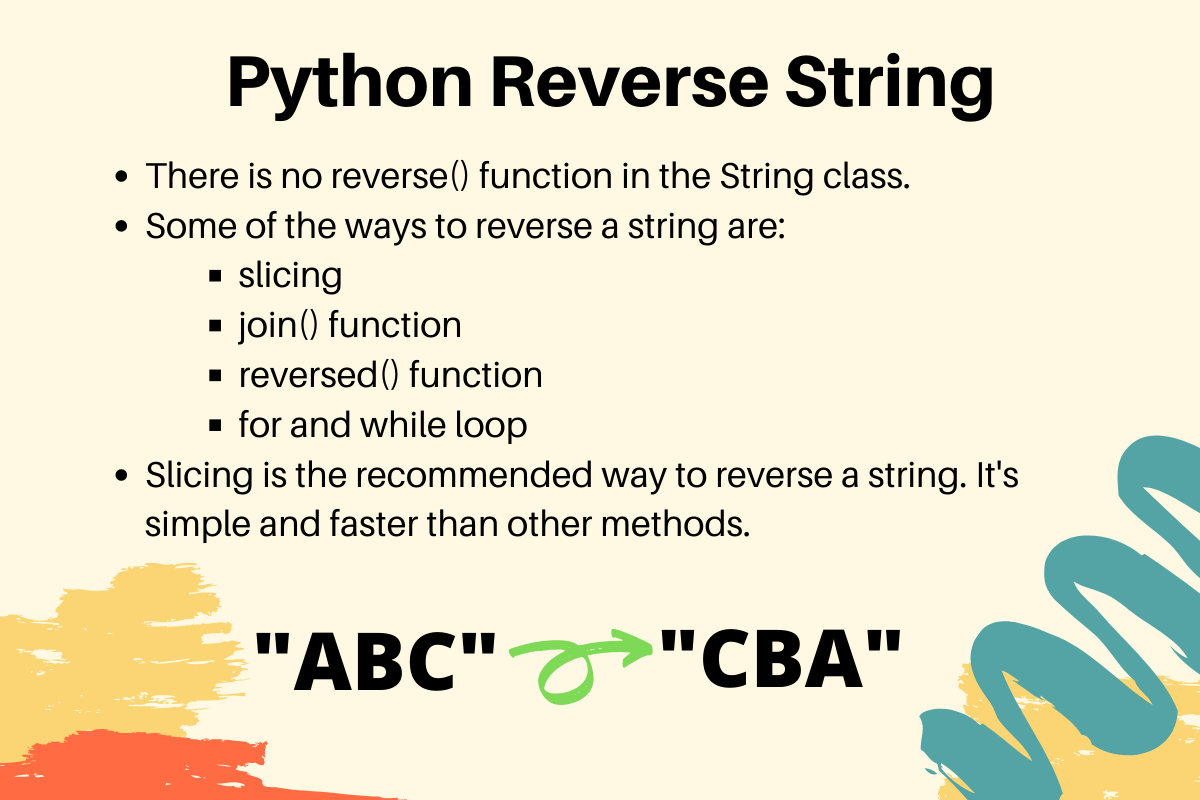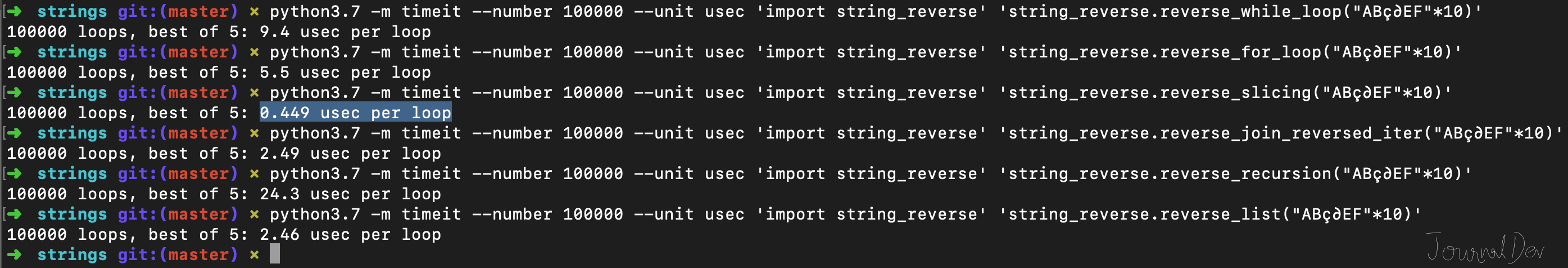# Python Reverse String - 5 Ways and the Best One

Published on August 3, 2022By PankajWhile we believe that this content benefits our community, we have not yet thoroughly reviewed it. If you have any suggestions for improvements, please let us know by clicking the “report an issue“ button at the bottom of the tutorial.

Python String doesn’t have a built-in reverse() function. However, there are various ways to reverse a string in Python.

## 1. How to Reverse a String in Python?

Some of the common ways to reverse a string are:

• Using Slicing to create a reverse copy of the string.
• Using for loop and appending characters in reverse order
• Using while loop to iterate string characters in reverse order and append them
• Using string join() function with reversed() iterator
• Creating a list from the string and then calling its `reverse()` function
• Using Recursion

### 1.1) Python Reverse String using Slicing

``````def reverse_slicing(s):
return s[::-1]

input_str = 'ABç∂EF'

if __name__ == "__main__":
print('Reverse String using slicing =', reverse_slicing(input_str))
``````

If you run above Python script, the output will be:

``````Reverse String using slicing = FE∂çBA
``````

### 1.2) Reverse String using For Loop

``````def reverse_for_loop(s):
s1 = ''
for c in s:
s1 = c + s1  # appending chars in reverse order
return s1

input_str = 'ABç∂EF'

if __name__ == "__main__":
print('Reverse String using for loop =', reverse_for_loop(input_str))
``````

Output: `Reverse String using for loop = FE∂çBA`

### 1.3) Reverse a String using While Loop

``````def reverse_while_loop(s):
s1 = ''
length = len(s) - 1
while length >= 0:
s1 = s1 + s[length]
length = length - 1
return s1

input_str = 'ABç∂EF'

if __name__ == "__main__":
print('Reverse String using while loop =', reverse_while_loop(input_str))
``````

### 1.4) Reverse a String using join() and reversed()

``````def reverse_join_reversed_iter(s):
s1 = ''.join(reversed(s))
return s1
``````

### 1.5) Python Reverse String using List reverse()

``````def reverse_list(s):
temp_list = list(s)
temp_list.reverse()
return ''.join(temp_list)
``````

### 1.6) Python Reverse String using Recursion

``````def reverse_recursion(s):
if len(s) == 0:
return s
else:
return reverse_recursion(s[1:]) + s
``````

## 2. Best Way to Reverse a String in Python

We can reverse a string through multiple algorithms. We have already seen six of them. But which of them you should choose to reverse a string. We can use timeit module to run multiple iterations of these functions and get the average time required to run them. All the above functions are stored in a python script named `string_reverse.py`. I executed all these functions one by one for 1,00,000 times using the timeit module and got the average of the best 5 runs.

``````\$ python3.7 -m timeit --number 100000 --unit usec 'import string_reverse' 'string_reverse.reverse_slicing("ABç∂EF"*10)'
100000 loops, best of 5: 0.449 usec per loop

\$ python3.7 -m timeit --number 100000 --unit usec 'import string_reverse' 'string_reverse.reverse_list("ABç∂EF"*10)'
100000 loops, best of 5: 2.46 usec per loop

\$ python3.7 -m timeit --number 100000 --unit usec 'import string_reverse' 'string_reverse.reverse_join_reversed_iter("ABç∂EF"*10)'
100000 loops, best of 5: 2.49 usec per loop

\$ python3.7 -m timeit --number 100000 --unit usec 'import string_reverse' 'string_reverse.reverse_for_loop("ABç∂EF"*10)'
100000 loops, best of 5: 5.5 usec per loop

\$ python3.7 -m timeit --number 100000 --unit usec 'import string_reverse' 'string_reverse.reverse_while_loop("ABç∂EF"*10)'
100000 loops, best of 5: 9.4 usec per loop

\$ python3.7 -m timeit --number 100000 --unit usec 'import string_reverse' 'string_reverse.reverse_recursion("ABç∂EF"*10)'
100000 loops, best of 5: 24.3 usec per loop
``````The below table presents the results and slowness of an algorithm from the best one.

Algorithm TimeIt Execution Time (Best of 5) Slowness
Slicing 0.449 usec 1x
List reverse() 2.46 usec 5.48x
reversed() + join() 2.49 usec 5.55x
for loop 5.5 usec 12.25x
while loop 9.4 usec 20.94x
Recursion 24.3 usec 54.12x

## 3. Summary

We should use slicing to reverse a string in Python. Its code is very simple and small and we don’t need to write our own logic to reverse the string. Also, it’s the fastest way to reverse a string as identified by the above test executions.

You can checkout complete python script and more Python examples from our GitHub Repository.

## 4. References

Thanks for learning with the DigitalOcean Community. Check out our offerings for compute, storage, networking, and managed databases.Pankaj

author

#### Still looking for an answer?

Ask a questionSearch for more help

JournalDev
DigitalOcean EmployeeDecember 8, 2021

Hi, Thank you for the explanation. I also wanted to know how to add new lines to the output. For example I have made a reverse string input program: def reverse_slice(s): return s[::-1] s=input('enter a string ') print(s[::-1]) How would I a make my answer be on multiple lines?

- J

JournalDev
DigitalOcean EmployeeMarch 21, 2020

Hi, Could you please help to explain the logic, as in what does Python do, for 1.1) Python Reverse String using Slicing. Confusing part are the double colon operators. I understand the first one is to tell Python to look at all the elements in string, the second one I’m unsure about but [ -1] means that the first item to return needs to be at length [-1]. What does the second colon operator tell Python to do? Thanks

- Kai

JournalDev
DigitalOcean EmployeeMarch 7, 2020

great content thanks

- anonymus

JournalDev
DigitalOcean EmployeeMay 14, 2019

Hi Pankaj, very useful indeed. I have a question: Why is this piece of code picking the character in the last position of the string and not the char in the position zero of the string? (if I move the char operand in the assignment statement to the other side of the operator then Python picks the char in position zero) s1 = c + s1 # appending chars in reverse order Thanks for clarifying. Rody

- Rody

JournalDev
DigitalOcean EmployeeMay 2, 2019